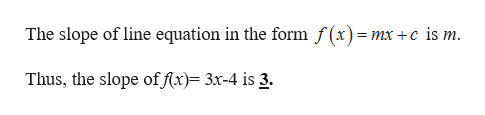# compute the slope to each of the following lines(A) f(x)= 3x-4(B) 2x-5y=20

Question
2 views

compute the slope to each of the following lines

(A) f(x)= 3x-4

(B) 2x-5y=20

check_circle

Step 1

(A) Given equation is f(x)= 3x-4.

...help_outlineImage TranscriptioncloseThe slope of line equation in the form f(x)=mx +c is m. Thus, the slope of f(x) 3x-4 is 3. fullscreen

### Want to see the full answer?

See Solution

#### Want to see this answer and more?

Solutions are written by subject experts who are available 24/7. Questions are typically answered within 1 hour.*

See Solution
*Response times may vary by subject and question.
Tagged in

### Algebra